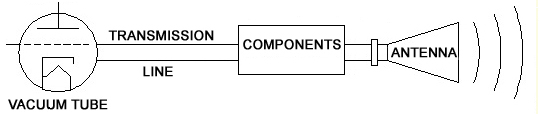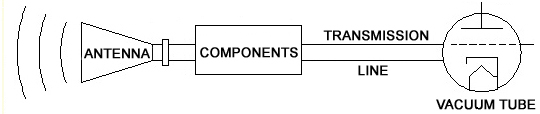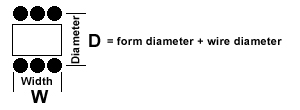# RF Calculators

## Decibel (dBm) to Watts Converter (and back)

The decibel to Watts converter lets you convert from dBm to Watts or the reverse; from Watts to dBm. It also supports dbW, kilowatts, megawatts and milliwatts.

 dBm dBW MW KW W PdBm = 10 * log10(PW) + 30 PdBW = 10 * log10(PW) PW = 10((PdBm-30)/10) 1 MW = 1000 KW = 1,000,000 W

In the world of RF energy, power levels can vary enormously. Instead of babysitting all of those zeros in calculations and tables, the power is converted to decibels. Decibels handle the zeros in a much tidier way. These units are abbreviated to dBW or abbreviated even further to dB. Sometimes the Watts are converted to milliwatts before they are converted to decibels. In this case, the units are referred to as dBm.

Decibels convey magnitude in a compact way, for example:

 dBW Watts dBm milliwatts These are all the same 10 10 40 10,000 These are all the same 30 1,000 60 1,000,000 These are all the same 70 10,000,000 100 10,000,000,000

## Wavelength to Frequency Converter (and back)

The wavelength to frequency calculator calculates frequency for a given wavelength and vice versa. The converter supports centimeters (cm), inches (in), feet (ft) or meters (m). Type in a number, press the Enter key and the converter will display your conversion.

 Wavelength ( λ ) cm in ft m Frequency ( f ) Hz KHz MHz GHz c = 299,792,458 m/sec λ = c / f f = c / λ

RF signals have a wavelength associated with them. Yes, that’s an actual physical length measurable in feet or meters. You might wonder how energy made of electric and magnetic fields can have a physical dimension.

Radio signals are made by devices that output oscillating voltages. The voltage rises to a positive peak, drops to a negative and then comes back to zero. That is one cycle. This happens over and over again, very quickly. The number of times this happens in a second is called the frequency (measured in Hertz). Antennas send this oscillating voltage out into the air where it travels at the speed of light (186,282 miles/second). Picture yourself flipping a rope and watching the wave travel away from you. That’s what’s happening, only much faster. The wavelength comes from looking at how far the wave travels in the time it takes to make one cycle. Here’s an example:

1GHz = 1,000,000,000 cycles/second or .000000001 seconds/cycle

Speed of light 186,282 miles/second x .000000001 seconds/cycle = .00186 miles/cycle = 11.8 inches/cycle

RF engineers use this dimension when they design RF circuits. The number lets them force the electricity to do certain things by controlling conductor dimensions.

## Field Strength Calculator

The field strength calculator will calculate Volts per meter (V/m) generated by a transmitter. The strength of the field is based on transmitter power, system loss and antenna gain.

## Transmitter→Field Strength

dB
dBV/m

This field strength calculator is the reverse of the one above. It will calculate the power required at the transmitter to result in a particular field strength. Power required at the transmitter is based on field strength, system loss and antenna gain.

## Field Strength →Transmitter

V/m
dB
dBElectromagnetic fields, as the name implies, have an electric field component and a magnetic field component. The two interact with each other in a way described by Maxwell’s equations, each field inspiring the other.

The electric field in front of an antenna is easier to measure than the magnetic field and is used more often to describe the field. The strength of an E (electric) field is expressed in Volts per meter (V/m). A measurement of 1V/m means there is 1 Volt of potential between two points that are one meter apart.

The field in front of an antenna propagates as a wave that can be described and modeled in several ways. One way is to describe it as a simple oscillating field. Another way is to describe it as energy delivered in packets called quanta. Physicists use both methods in their calculations.

## Capacitive Reactance Calculator

The calculator computes capacitive reactance based on frequency and capacitance. It supports Hertz (Hz), kilohertz (kHz), megahertz (MHz), gigahertz (GHz), picofarads (pF) and microfarads (uF).

GHz
pF
Ω

XC = 1 / ( 2πFC )

Reactance can be thought of as AC (alternating current) resistance. While a DC (direct current) will charge a capacitor to the value of its voltage source and stop, AC will see a capacitor as a resistive load. With AC, there will be a voltage drop across the capacitor and the current will be limited. The reactance of a capacitor will increase as frequency or capacitance decreases.

## Air Core Inductance Calculator

This calculator computes the inductance of a coil of wire (single layer) with an air core. The output is based on the width and diameter of the coil.Units Inches Centimeters µH

L = D2 x T2 / (18 D + 40 W)

Inductance is value describing how hard a device opposes a change in current. You can compare it to the inertia of a flywheel or a bicycle. It takes a push to get things moving and once they are moving they don’t want to stop. Current flowing through an inductor works the same way. Opening an electrical switch in a highly inductive circuit will cause an arc or spark because the current literally jumps through the air to keep flowing. How is this is possible? Energy storage.

Current moving through a wire inspires a magnetic field around the wire. Like a flywheel, it takes energy to get this magnetic field going. Once it is going, it doesn’t want to stop. The field is additive and by winding the wire into a cylindrical coil, the field is multiplied creating higher inductance. More turns; more inductance. The inductance can be increased further by winding the wire around iron or other materials.